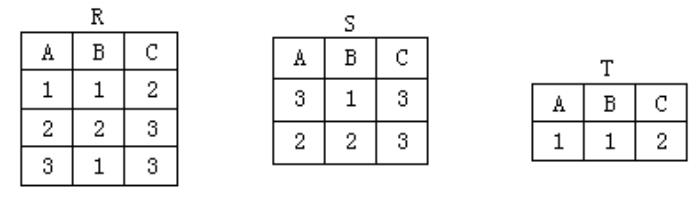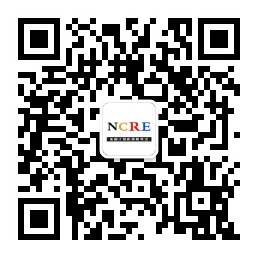400-8077-735

# NCRE等级考试《二级 月 C语言》考试题库二

9.有三个关系R、S和T如下A. T=R∩S

B. T=R∪S

C. T=R×S

D. T=R-S

【答案】:D

【解析】:

10.下列选项中，不属于数据管理员(DBA)职责的是（ ）。

A. 数据库维护

B. 数据库设计

C. 改善系统性能，提高系统效率

D. 数据类型转换

【答案】:D

【解析】:

11.有以下程序

#include<stdio.h>

main()

{

inta=0,b=0;

/*给a赋值a=10;

printf("a+b=%d\n",a+b);/*输出计算结果*/

}

A.a+b=0

B.a+b=30

C.a+b=10

D. 出错

【答案】:A

【解析】:

12.有以下程序段

#include <stdio.h>

main()

{

inta,b,c;

a=10;b=50;c=30;

if(a>b)a=b,b=c;c=a;

printf("a=%d b=%d c=%d\n",a,b,c);

}

A.a=10 b=50 c=30

B.a=10 b=50 c=10

C.a=10 b=30 c=10

D.a=50 b=30 c=50

【答案】:B

【解析】:

C语言中使用分号来作为语句的结束，所以a=b,b=c;是一条含有逗号运算符的语句，是if语句的执行体。因为题中a<b，if条件不满足，直接执行c=a。答案选择B选项。

13.下列二叉树描述中，正确的是（ ）。

A. 任何一棵二叉树必须有一个度为2的结点

B. 二叉树的度可以小于2

C. 非空二叉树有0个或1个根结点

D. 至少有2个根结点

【答案】:B

【解析】:

14.下列叙述中，不符合良好程序设计风格要求的是（ ）。

A. 程序的效率第一，清晰第二

B. 程序的可读性好

C. 程序中要有必要的注释

D. 输入数据前要有提示信息

【答案】:A

【解析】:

“清晰第一，效率第二”是当今主导的程序设计风格。对程序的要求不仅是能够运行正常，还要便于调试和维护，所以程序要具有良好的可读性，语句结构应该简单直接，这有利于程序的开发与维护。答案选择A选项。

15.有以下程序

#include <stdio.h>

main()

{

int x,y,z;

x=y=1;

z=x++,y++,++y;

printf("%d,%d,%d\n",x,y,z);

}

A. 2,3,1

B. 2,3,2

C. 2,3,3

D. 2,2,1

【答案】:A

【解析】:《计算机等级考试网》免责声明：

1、因考试政策、内容不断变化与调整，本网站提供的以上信息仅供参考，如有异议，请考生以权威部门公布的内容为准！

2、本网信息来源为其他媒体的稿件转载，免费转载出于非商业性学习目的，版权归原作者所有，如有内容与版权问题等请与本站联系。联系邮箱：812379481@qq.com。

### 互动交流

• ###### 扫码进入微信刷题助手解锁即可开始刷题

并加入考生交流群

• ###### 扫码加入微信交流群与广大计算机等级考生

共同学习交流